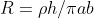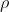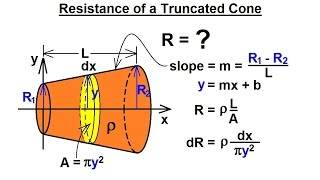# Finding the voltage drop along a tapered wire

• Sorin2225
In summary, the conversation is about finding the voltage of a tapered wire with different radii at the two ends. The formula discussed is R = ρh/πab or ∫dR = ∫(ρ/A(l))dl, which could be derived using calculus. It could represent the resistance of a tapered conductor or a conductor with an elliptical cross section. The origin of the expression is not clear, but it is suggested that it may be related to the "equal areas equal times" concept for an elliptical Keplerian orbit. f

#### Sorin2225

Homework Statement
This isn't my actual homework question per se, I came upon this formula and I'm curious to know where it comes from.
Relevant Equations
R = p*h/pi*a*b and R = P*h/A
The actual homework question is about finding the voltage of a tapered wire, one where the two ends are different sizes (1cm and 2cm in this case). I've been searching for an equation to do this simply by just plugging in the values I have and I came across this.Whereis resistivity, h is length of wire and a and b a radii of two cross sections
I've familiar with ph/A or pl/A but I've don't think I've ever seen it with Pi*a*b replace the A. I was wondering if anyone could just explain if this is in some book or it if it is derived from something else.

Thanks

##\pi a b## seems like the area of an elliptical cross section of semi-major/minor axes of a and b.

Can you be more specific regarding the origin of the expression?

•erobz
I don't know what that formula is for, but I think instead you are going to want to use:

$$\int dR = \int \frac{ \rho}{A(l)} dl$$

Last edited:
•Delta2, bob012345 and Orodruin
Homework Statement:: This isn't my actual homework question per se, I came upon this formula and I'm curious to know where it comes from.
Relevant Equations:: R = p*h/pi*a*b and R = P*h/A

The actual homework question is about finding the voltage of a tapered wire, one where the two ends are different sizes (1cm and 2cm in this case). I've been searching for an equation to do this simply by just plugging in the values I have and I came across this.
View attachment 304266
Where View attachment 304267 is resistivity, h is length of wire and a and b a radii of two cross sections
I've familiar with ph/A or pl/A but I've don't think I've ever seen it with Pi*a*b replace the A. I was wondering if anyone could just explain if this is in some book or it if it is derived from something else.

Thanks
Have you had Calculus yet? That is how this formula was derived as @erobz showed.

•erobz
##\pi a b## seems like the area of an elliptical cross section of semi-major/minor axes of a and b.

Can you be more specific regarding the origin of the expression?
I actually just did the integration (I suspect you were already aware of this...I was not). Turns out the formula wasn't an error, just not well described.

The actual homework question is about finding the voltage of a tapered wire
You need to accurately state the complete question. E.g. what is the current? Between what points (presumably the ends?) is the voltage measured?

, one where the two ends are different sizes (1cm and 2cm in this case)

I've been searching for an equation to do this simply by just plugging in the values
You are probably expected to derive the formula for yourself.

I have and I came across this.
View attachment 304266
Where View attachment 304267 is resistivity, h is length of wire and a and b a radii of two cross sections
I've familiar with ph/A or pl/A but I've don't think I've ever seen it with Pi*a*b replace the A. I was wondering if anyone could just explain if this is in some book or it if it is derived from something else.
I'd guess you are meant to derive a formula yourself, using some calculus. That is probably the main part of the exercise which earns you most of the marks.

Have you had Calculus yet? That is how this formula was derived as @erobz showed.
I had no idea we were actually ending up there (doesn't matter, but just an FYI)!Last edited:
$$\int dR = \int \frac{ \rho}{A} dl$$
That works and gives the desired answer. One has to be careful to write ##A## in the denominator as a function of the linear variable ##l##.

On edit:
The expression ##R=\dfrac{\rho h}{\pi ab}~## could be the resistance of a tapered conductor of length h and radii ##a## and ##b##. When ##a=b## (no taper) the formula correctly reduces to the expression for a cylindrical conductor.

However, it could also be the resistance of a conductor of uniform elliptical cross section of semi major and minor axes ##a## and ##b##. I don't have an intuitive explanation for this, other than "because it comes out of the math". Is the explanation obvious to someone else?

Last edited:
•bob012345 and erobz
I think the reason will look a lot like "equal areas equal times" for an elliptical Keplerian oorbit. But that gives me scant insight even if true.

Thanks for the help everyone, like I said I did just kind of stumble upon the equation as someone else was using it to solve a similar question. Sorry if it wasn't described well. Also sorry for not putting in the values needed but I didn't want anyone to solve anything, just wanted to know if the equation was derived from somewhere.

•bob012345
That works and gives the desired answer. One has to be careful to write ##A## in the denominator as a function of the linear variable ##l##.

On edit:
The expression ##R=\dfrac{\rho h}{\pi ab}~## could be the resistance of a tapered conductor of length h and radii ##a## and ##b##. When ##a=b## (no taper) the formula correctly reduces to the expression for a cylindrical conductor.

However, it could also be the resistance of a conductor of uniform elliptical cross section of semi major and minor axes ##a## and ##b##. I don't have an intuitive explanation for this, other than "because it comes out of the math". Is the explanation obvious to someone else?
I don't have an intuitive explanation as such but I noticed that if the taper is linear, and the resistance ##R## is of the form $$R = \frac{\rho h}{A_{eff}}$$ the effective area seems to be just the geometric mean of the end area's which in this case is;

$$A_{eff} = \left( \pi a^2 \pi b^2 \right)^{\frac{1}{2}} = \pi a b$$

It could also work for a circle of radius ##r= \sqrt{ab}## or a rectangle of sides ##\pi a## and ##b## ect. or any shape with total area ##\pi a b## so there would be an infinite number of shapes for the same area and thus the same resistance. I integrated a square taper from ##a## to ##b## and got $$R = \frac{\rho h}{a b}$$

I suspect that for any linearly tapered resistor of end area's ##A_1## and ##A_2## of the same shape, the effective area is

$$A_{eff} = \left( A_1 A_2 \right)^{\frac{1}{2}}$$

•erobz
if the taper is linear
The area tapers linearly or the linear dimension tapers linearly?

The area tapers linearly or the linear dimension tapers linearly?
The linear dimension as in the original case with radius ##a## going to radius ##b## or the square case where the edge length goes from ##a## to ##b## linearly. The line of increasing/decreasing taper is along the length of the resistor so it looks like a cone.Last edited:
as in the original case
The original question is
finding the voltage of a tapered wire, one where the two ends are different sizes (1cm and 2cm in this case)
It is not clear that it is even linear, let alone whether any such linearity is wrt radius or area. It goes on to quote an expression which might or might not be relevant, but for which one can deduce the assumption.

I suspect that for any linearly tapered resistor of end area's ##A_1## and ##A_2## of the same shape, the effective area is

$$A_{eff} = \left( A_1 A_2 \right)^{\frac{1}{2}}$$
Consider rectangular end areas with side lengths ##(a,b)## and ##(b,a)##, respectively. Both will clearly have area ##A = ab##.

The linear tapering of each dimension implies that ##x = kz + a## and ##y = b - kz##, where ##k = (b - a)\ell##, ##x## and ##y## being the side lengths of the rectangular area and ##z## the coordinate along the resistor. This results in
$$R = \int_0^\ell \frac{\rho}{xy}\, dz = \rho \int_0^\ell \frac{dz}{(kz + a)(b - kz)} = \frac{\rho}{a+b} \int_0^\ell \left[\frac{1}{kz+a} + \frac{1}{b-kz}\right]dz = \frac{\rho}{k(a+b)} \left[\ln\left(\frac{kz + a}{b - kz}\right)\right]_0^\ell = \frac{\rho}{k(a+b)} \ln\left[\frac{b}{a} \frac{b}{a}\right] = \frac{2\rho \ell}{b^2 - a^2} \ln(b/a).$$
... assuming I did the integrals correctly. So the geometric mean seems to apply only if the area scaling quadratically rather than each dimension scaling linearly.

The original question is

It is not clear that it is even linear, let alone whether any such linearity is wrt radius or area. It goes on to quote an expression which might or might not be relevant, but for which one can deduce the assumption.
The OP was really asking about where the formula ##R= \frac{\rho h}{\pi a b}## came from, not the homework question about voltage that was referenced. The ensuing discussion as about that and that is what I meant by original case.
Consider rectangular end areas with side lengths ##(a,b)## and ##(b,a)##, respectively. Both will clearly have area ##A = ab##.
This is already confusing. Two separate rectangles? The two ends of one tapered resistor? What do you mean?

This is already confusing. Two separate rectangles? The two ends of one tapered resistor? What do you mean?
The end areas being rectangles of the same size, but with 90 degree difference in orientation. The side lengths scaling linearly.

... assuming I did the integrals correctly. So the geometric mean seems to apply only if the area scaling quadratically rather than each dimension scaling linearly.

Consider rectangular end areas with side lengths ##(a,b)## and ##(b,a)##, respectively. Both will clearly have area ##A = ab##.

The linear tapering of each dimension implies that ##x = kz + a## and ##y = b - kz##, where ##k = (b - a)\ell##, ##x## and ##y## being the side lengths of the rectangular area and ##z## the coordinate along the resistor. This results in
$$R = \int_0^\ell \frac{\rho}{xy}\, dz = \rho \int_0^\ell \frac{dz}{(kz + a)(b - kz)} = \frac{\rho}{a+b} \int_0^\ell \left[\frac{1}{kz+a} + \frac{1}{b-kz}\right]dz = \frac{\rho}{k(a+b)} \left[\ln\left(\frac{kz + a}{b - kz}\right)\right]_0^\ell = \frac{\rho}{k(a+b)} \ln\left[\frac{b}{a} \frac{b}{a}\right] = \frac{2\rho \ell}{b^2 - a^2} \ln(b/a).$$
... assuming I did the integrals correctly. So the geometric mean seems to apply only if the area scaling quadratically rather than each dimension scaling linearly.
I think you are doing a twisted shape? In both cases I mentioned the shape was the same yet increased along the linear dimension in both x and y-axis but at the same rate so the areas do scale quadratically. For an arbitrary shape, if you scale linearly in x and y like space is expanding it changes the area quadratically. That was built into my arguments whether so sorry if I did not made it clear.

I think you are doing a twisted shape?
No. Each cross section is a rectangle and the sides are flat.

In both cases I mentioned the shape was the same yet increased along the linear dimension in both x and y-axis but at the same rate so the areas do scale quadratically.
As I said, the important thing here is area scaling quadratically, which it does under your assumption.

So the geometric mean seems to apply only if the area scaling quadratically rather than each dimension scaling linearly.

The end areas being rectangles of the same size, but with 90 degree difference in orientation. The side lengths scaling linearly.
Shouldn't ##k =\large \frac{b-a}{l}##? You have ##k = (b-a)l##

Shouldn't ##k =\large \frac{b-a}{l}##? You have ##k = (b-a)l##
Yes, that is just a typo. I used the correct expression in the rest of the post.

Oh, and as always, it is good to check your limiting cases. When ##b = a+\epsilon## we obtain to linear order in ##\epsilon##:

$$R = \frac{2\rho \ell}{a^2 + 2a\epsilon -a^2} \ln{1 + \epsilon/a) = \frac{\rho \ell}{a\epsilon} \frac{\epsilon}{a} = \frac{\rho \ell}{a^2},$$
which at least is the correct limit.

Yes, that is just a typo. I used the correct expression in the rest of the post.
Implicit in my argument was the idea that the shape was fixed, it just got bigger or smaller. Your case is an interesting one but I would not call it a tapered resistor. I would call it a funky twisting psychedelic resistor.It is not twisting.

Each side is tapered linearly, just in different directions.

More like a transformation than a twisting then. As an aside, if one rotated the rectangle smoothly 90° (or any angle) over the length I would expect a constant resistance since each differential volume would have the same area.

More like a transformation than a twisting then. As an aside, if one rotated the rectangle smoothly 90° (or any angle) over the length I would expect a constant resistance since each differential volume would have the same area.
It could be a lot more complicated than that.
If we assume the current density is the same everywhere then the twisting has increased the path length and the effective cross section (normal to the current) has reduced.
If not, it's really messy.

It could be a lot more complicated than that.
If we assume the current density is the same everywhere then the twisting has increased the path length and the effective cross section (normal to the current) has reduced.
If not, it's really messy.
This is true also for the tapered wire where the cross section keeps its shape. The underlying working assumption throughout here has been that those effects are small.

This is true also for the tapered wire where the cross section keeps its shape. The underlying working assumption throughout here has been that those effects are small.
They'd be small for an untwisted wire as long as its length >> width, but for a highly twisted wire of square cross section the path length increase under the constant density model would not be so small. Hard to say what would happen in the truer model. In the limit, the effective cross section reduces from ##a^2## to ##\frac \pi 4a^2##.

They'd be small for an untwisted wire as long as its length >> width, but for a highly twisted wire of square cross section the path length increase under the constant density model would not be so small.
Well, obviously, but nobody specified the wire to be heavily twisted or the tapered wire to be long (although that may be implicit in ”wire”).

nobody specified the wire to be heavily twisted
No, I was a bit off topic, but responding to post #25.

It could be a lot more complicated than that.
If we assume the current density is the same everywhere then the twisting has increased the path length and the effective cross section (normal to the current) has reduced.
If not, it's really messy.
In the truncated conical resistor problem above we calculated the total resistance under the assumption that a simple integration over the geometry yields the correct formula and answered the OP's question of where the formula came from.

But here is a paper that examines the physics of current flow and potential in such a resistor by authors Romano and Price from the University of Utah. Enjoy. The introduction reads;

ABSTRACT
A truncated cone, made of material of uniform resistivity, is given in many introductory physics texts as a nontrivial problem in the computation of resistance. The intended method and answer are incorrect and the problem cannot be solved by elementary means. In this paper, we (i) discuss the physics of current flow in a non-constant cross‐section conductor, (ii) examine the flaws in the ‘‘standard’’ solution for the truncated cone, (iii) present a computed resistance found from a numerically generated solution for the electrical potential in the truncated cone, and (iv) consider whether any problem exists to which the standard solution applies.
1. © 1996 American Association of Physics Teachers.
https://www.researchgate.net/publication/252273146_The_conical_resistor_conundrum_A_potential_solution

Last edited: